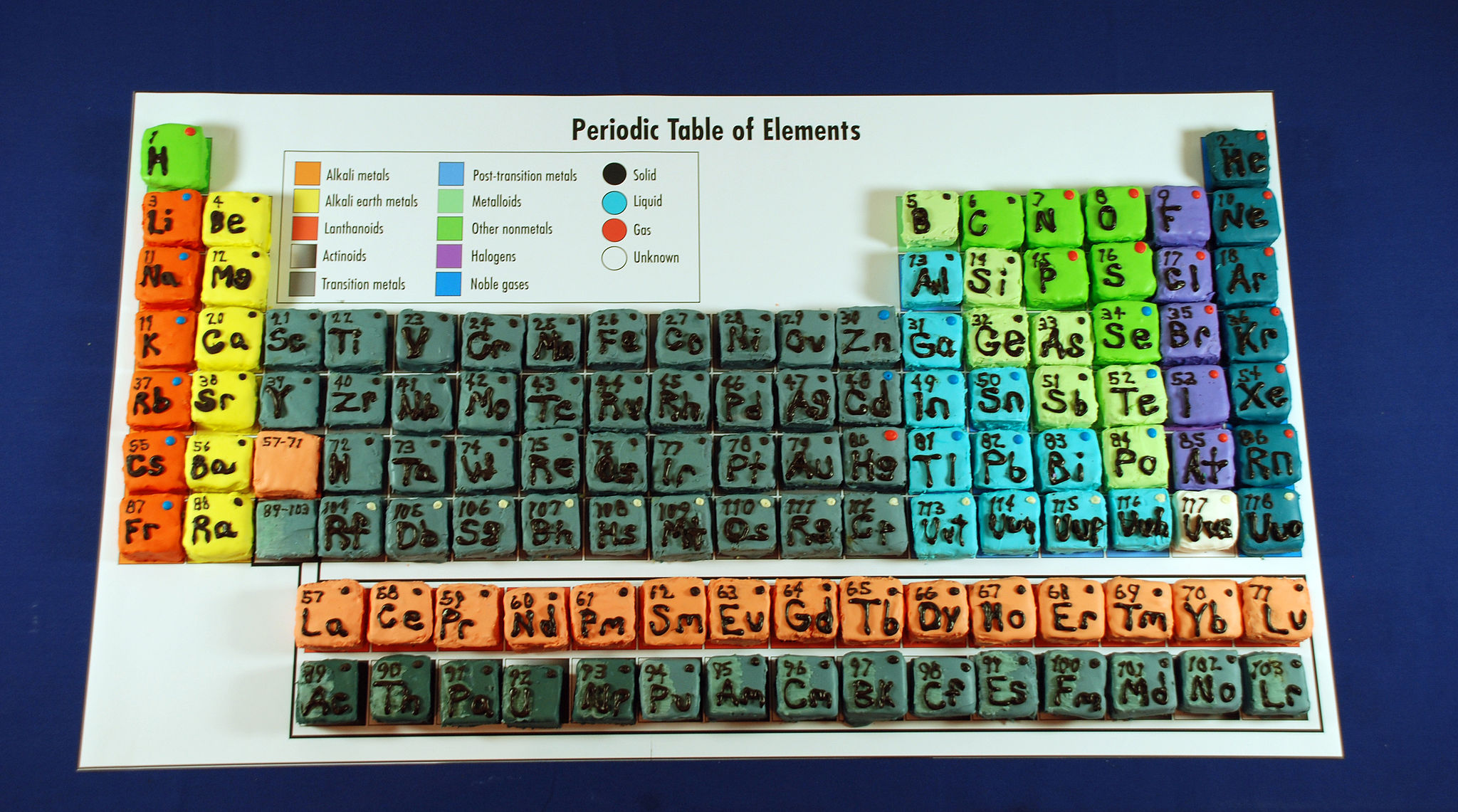# Periodic table of elements

Since the periodic table of elements in brilliant wiki is blurred and not very useful, I shall remake it here :)

#### Physic constants:

Light speed in a vacuum: $299792458$ meters per second

#### Something fun:Cupcakes from the first-anniversary celebration of the Chemical Heritage Foundation's museum. Photographer: Conrad Erb. Creative Commons license for reuse and modification.

#### Periodic table of elements:

Legend: $\color{#3D99F6}\text{other nonmetals},\color{7f7f00} \text{alkali metals},\color{ff7f9f}\text{alkaline earth metals},\color{7f7f7f}\text{transition metals},\color{2f2fff}\text{noble gases},\color{#D61F06}\text{post-transition metals},\color{ff2faf}\text{metalloids},$
$\color{#20A900}\text{halogens},\color{8f008f}\text{lanthanides},\color{008f6f}\text{actinides},\color{#333333}\text{unknown properties}$
$\colorbox{00ffff}{\text{gas}},\colorbox{ffcfff}{\text{solid}},\colorbox{ffff00}{\text{liquid}},\fbox{\text{unknown}}$

 Group Period 1- IA 2- IIA 3- IIIB 4- IVB 5- VB 6- VIB 7- VIIB 8- VIII 9- VIII 10- VIII 11- IB 12- IIB 13- IIIA 14- IVA 15- VA 16- VIA 17- VIIA 18- VIIIA 1 $\scriptsize\color{#3D99F6}\begin{matrix}1~~\colorbox{00ffff}{\text{H}}\\ \text{Hydrogen}\\1.0078\end{matrix}$ $\scriptsize\color{2f2fff}\begin{matrix}2~~\colorbox{00ffff}{\text{He}}\\ \text{Helium}\\4.0026\end{matrix}$ 2 $\scriptsize\color{7f7f00}\begin{matrix}3~~\colorbox{ffcfff}{\text{Li}}\\ \text{Lithium}\\6.941\end{matrix}$ $\scriptsize\color{ff7f9f}\begin{matrix}4~~\colorbox{ffcfff}{\text{Be}}\\ \text{Beryllium}\\9.0122\end{matrix}$ $\scriptsize\color{ff2f8f}\begin{matrix}5~~\colorbox{ffcfff}{\text{B}}\\ \text{Boron}\\10.806\end{matrix}$ $\scriptsize\color{#3D99F6}\begin{matrix}6~~\colorbox{ffcfff}{\text{C}}\\ \text{Carbon}\\12.009\end{matrix}$ $\scriptsize\color{#3D99F6}\begin{matrix}7~~\colorbox{00ffff}{\text{N}}\\ \text{Nitrogen}\\14.006\end{matrix}$ $\scriptsize\color{#3D99F6}\begin{matrix}8~~\colorbox{00ffff}{\text{O}}\\ \text{Oxygen}\\15.999\end{matrix}$ $\scriptsize\color{#20A900}\begin{matrix}9~~\colorbox{00ffff}{\text{F}}\\ \text{Fluorine}\\18.998\end{matrix}$ $\scriptsize\color{2f2fff}\begin{matrix}10~~\colorbox{00ffff}{\text{Ne}}\\ \text{Neon}\\20.180\end{matrix}$ 3 $\scriptsize\color{7f7f00}\begin{matrix}11~~\colorbox{ffcfff}{\text{Na}}\\ \text{Sodium}\\22.990\end{matrix}$ $\scriptsize\color{ff7f9f}\begin{matrix}12~~\colorbox{ffcfff}{\text{Mg}}\\ \text{Magnesium}\\24.305\end{matrix}$ $\scriptsize\color{#D61F06}\begin{matrix}13~~\colorbox{ffcfff}{\text{Al}}\\ \text{Aluminium}\\26.983\end{matrix}$ $\scriptsize\color{ff2f8f}\begin{matrix}14~~\colorbox{ffcfff}{\text{Si}}\\ \text{Silicon}\\28.084\end{matrix}$ $\scriptsize\color{#3D99F6}\begin{matrix}15~~\colorbox{ffcfff}{\text{P}}\\ \text{Phosphorus}\\30.974\end{matrix}$ $\scriptsize\color{#3D99F6}\begin{matrix}16~~\colorbox{ffcfff}{\text{S}}\\ \text{Sulfur}\\32.059\end{matrix}$ $\scriptsize\color{#20A900}\begin{matrix}17~~\colorbox{ffcfff}{\text{Cl}}\\ \text{Chlorine}\\35.446\end{matrix}$ $\scriptsize\color{2f2fff}\begin{matrix}18~~\colorbox{00ffff}{\text{Ar}}\\ \text{Argon}\\39.948\end{matrix}$ 4 $\scriptsize\color{7f7f00}\begin{matrix}19~~\colorbox{ffcfff}{\text{K}}\\ \text{Potassium}\\39.098\end{matrix}$Note by Jeff Giff
4 months, 2 weeks ago

This discussion board is a place to discuss our Daily Challenges and the math and science related to those challenges. Explanations are more than just a solution — they should explain the steps and thinking strategies that you used to obtain the solution. Comments should further the discussion of math and science.

When posting on Brilliant:

• Use the emojis to react to an explanation, whether you're congratulating a job well done , or just really confused .
• Ask specific questions about the challenge or the steps in somebody's explanation. Well-posed questions can add a lot to the discussion, but posting "I don't understand!" doesn't help anyone.
• Try to contribute something new to the discussion, whether it is an extension, generalization or other idea related to the challenge.

MarkdownAppears as
*italics* or _italics_ italics
**bold** or __bold__ bold
- bulleted- list
• bulleted
• list
1. numbered2. list
1. numbered
2. list
Note: you must add a full line of space before and after lists for them to show up correctly
paragraph 1paragraph 2

paragraph 1

paragraph 2

[example link](https://brilliant.org)example link
> This is a quote
This is a quote
    # I indented these lines
# 4 spaces, and now they show
# up as a code block.

print "hello world"
# I indented these lines
# 4 spaces, and now they show
# up as a code block.

print "hello world"
MathAppears as
Remember to wrap math in $$ ... $$ or $ ... $ to ensure proper formatting.
2 \times 3 $2 \times 3$
2^{34} $2^{34}$
a_{i-1} $a_{i-1}$
\frac{2}{3} $\frac{2}{3}$
\sqrt{2} $\sqrt{2}$
\sum_{i=1}^3 $\sum_{i=1}^3$
\sin \theta $\sin \theta$
\boxed{123} $\boxed{123}$

Sort by:

Why are you making a LaTeX one? A picture would be much more efficient tbh...

- 4 months, 2 weeks ago

Umm... I just like it this way :)

- 4 months, 2 weeks ago

Ok :)

- 4 months, 2 weeks ago

Besides, I’m including the table in Randall Munroe(creator of xkcd)’s Thing Explainer.

- 4 months, 2 weeks ago